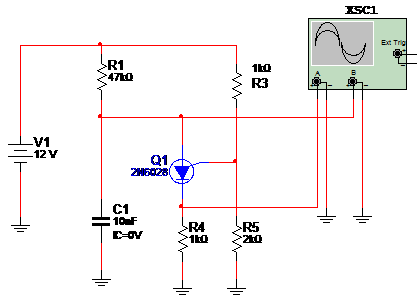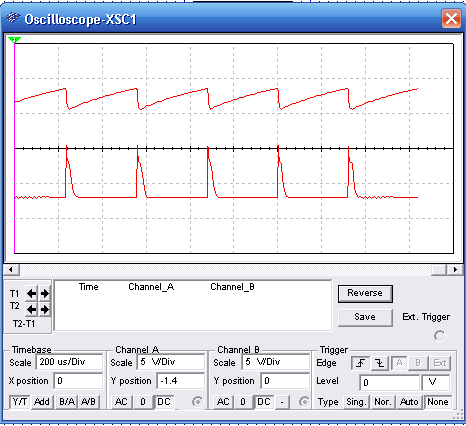## Relaxation Oscillator Using PUT (MSB075E)

This is a simulation of a Relaxation Oscillator using a programmable unijunction transistor (PUT). The circuit is as a oscillator using a UJT with the difference that R3 and R5 determines the trigger point of the transistor. In the simulation the oscilloscope shows the wave shapes in two points of the circuit. Figure 1 shows the circuit for the simulation.Figure 1 – Circuit for the simulation

Wave shapes in the circuit as displayed in the virtual oscilloscope of the MultiSIM are in figure 2.Figure 2 – Wave shapes in the circuit